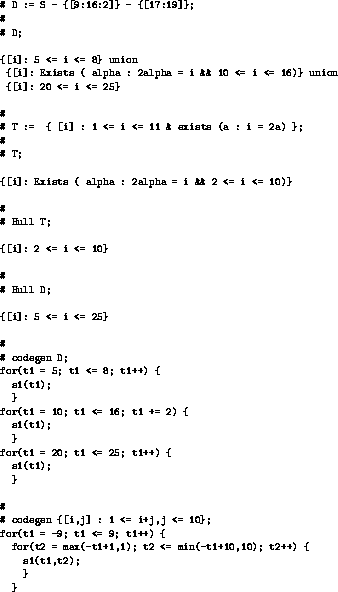Next: 3 Relational Expression syntax Up: The Omega Calculator Previous: 1 Introduction

# 2 Omega Calculator invocation, syntax and semantics

The calculator reads from the file given as the first argument, or standard input, and prints results on standard output. You can specify several command line flags. Using -D mk, where m is a character and k is a digit, sets the debugging level to k for module m. Using -Gg sets the maximum number of inequalities in a conjunct to g. Using -Ee sets the maximum number of equalities in a conjunct to E. In version 1.0, we intend to change our data structures so that these will not need to be specified. There is also a limit on the maximum number of variables in a conjunct, but this cannot be changed at run-time. It is given by maxVars in oc.h. We also intend to make this go away.

The input is a series of statements terminated by semicolons. A # indicates that the rest of the line is a comment. The syntax << filename >> can occur anywhere (and indicates that the text of the file is to be included here. The statements can have one of the forms listed in Figure 1. RelExpr is a short form of Relational Expression.Figure 1: Omega Calculator statements

Figures 2 and 2 show a sample session with the Omega Calculator. Lines starting with # are input to the Omega calculator, the other lines are output from the calculator.Figure 2: Example of the Omega Calculator in action: part 1Figure 3: Example of the Omega Calculator in action: part 2

Some relational operations may not preserve the names of input and output variables. If this happens, the variables get default names: In_n for input variables and Out_n for output variables, where n is a position of variables in its tuple. When printing, primes are added to variables to distinguish between multiple variables with the same name. In input, primes may also be used to distinguish between multiple variables with the same name: the primes are stripped before being passed to the Omega library.Next: 3 Relational Expression syntax Up: The Omega Calculator Previous: 1 Introduction

omega@cs.umd.edu

Web Accessibility